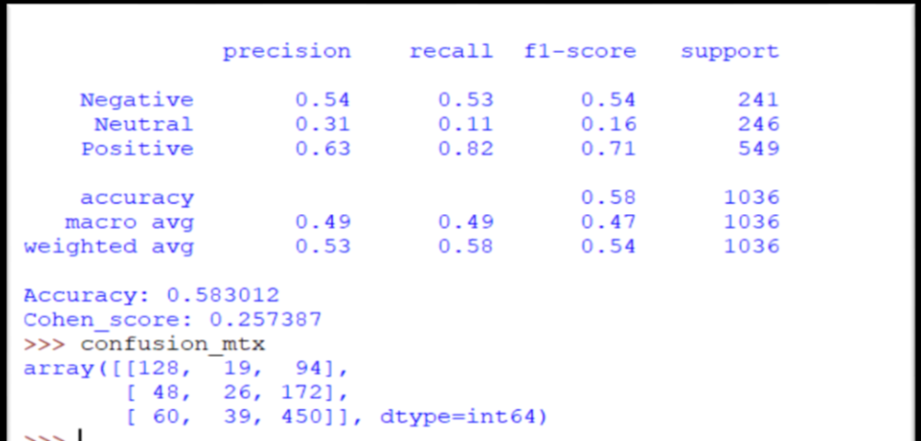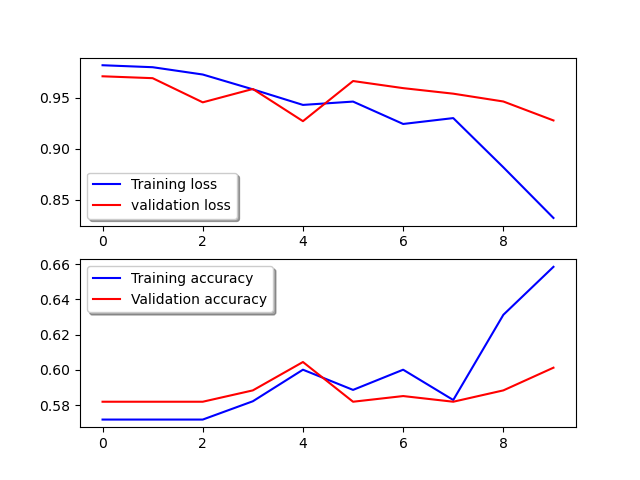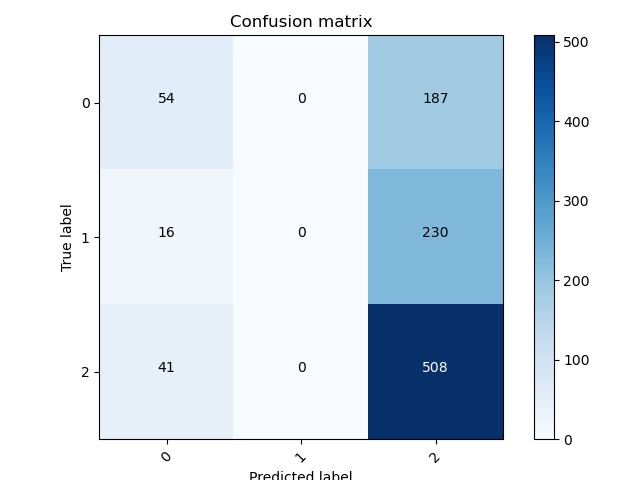# Deep learning based sentiment analysis of drug reviews## 1. Introduction

Finding the efficacy and usability of a medical drug as a cure for a disease can be a problem to solve as there are chances for side effects. Generalizable solution to this problem can be achieved by exploring the post-marketing drug surveillance methods such as drug reviews analysis to monitor drug-related issues arising after taking the medication. Based on the drug reviews of patients' feedback, our objective is to recognize the sentiment inclination (positive/negative/neutral) of drug reviews by performing sentiment analysis.

## 2. Objective

The proposed objective is to perform the sentiment analysis of drug reviews using deep learning techniques.

## 3. Dataset

Drug Review Dataset - The dataset provides patient reviews on specific drugs along with related conditions. Reviews and ratings are grouped into reports on the three aspects benefits, side effects and overall comment.

## 4. Methodology

The proposed methodology for building a deep learning model for sentiment analysis on drug reviews has the following steps:

### 4.1 Splitting of Data

• Data available in Train and Test tab seperated values (tsv) files
• Training model using hold-out.
• Train and Test set, following the 75-25% split ratio
• 90-10% split ratio of train dataset into train and validation set, for model training.

### 4.2 Importing packages



import string
import numpy as np
import pandas as pd
from numpy import argmax
from numpy import array
import re

import matplotlib.pyplot as plt
import matplotlib.image as mpimg
import seaborn as sns

from keras.preprocessing.text import Tokenizer
from keras.models import Sequential
from keras.layers import Dense, Flatten, LSTM, Conv1D, MaxPooling1D, Dropout, Activation
from keras.layers.embeddings import Embedding
from keras.utils.np_utils import to_categorical # convert to one-hot-encoding

import nltk
from nltk.corpus import stopwords
from nltk.stem.snowball import SnowballStemmer
from sklearn.metrics import cohen_kappa_score
from sklearn.metrics import accuracy_score
from sklearn.metrics import classification_report
from sklearn.model_selection import train_test_split
from sklearn.metrics import confusion_matrix
from sklearn.preprocessing import LabelEncoder
from sklearn.preprocessing import OneHotEncoder
import time
import itertools
import warnings
from sklearn.model_selection import StratifiedKFold



### 4.3 Data Exploration

For this purpose python pandas library is used to read the tsv files and load it in the working directory for further processing.



# Data Acquisition

#print("Train dataset shape :",Train_dataset.shape, " Test dataset shape: ",Test_dataset.shape,"\n")



### 4.4 Data Pre-processing

In this phase we will prepare our data for predicting patient satisfaction based on "rating" and "reviews". We will perform concatanation to join the "benifits_review", "side_effects_review" and "comments" into a "report" attribute to predict the overall satisfaction of the patients. Furthermore, labeling of ratings as Postive, Negative and Neutral for the sentiment classification.



# DATA PREPROCESSING Phase

# for Predicting Patient Satisfaction based on rating and all reviews

Prediction_Train_data = pd.DataFrame({'rating':Train_dataset.rating,
'benefits_reviews':Train_dataset.benefitsReview,
'side_effects_reviews':Train_dataset.sideEffectsReview,

Prediction_Test_data = pd.DataFrame({'rating':Test_dataset.rating,
'benefits_reviews':Test_dataset.benefitsReview,
'side_effects_reviews':Test_dataset.sideEffectsReview,

# performing concatanation to join benifits_review, side_effects_review and comments into a Report attribute to predict the overall satisfaction of the patients

Prediction_Train_data['report'] = Prediction_Train_data[report].apply(lambda row: '_'.join(row.values.astype(str)), axis=1)
Prediction_Test_data['report'] = Prediction_Test_data[report].apply(lambda row: '_'.join(row.values.astype(str)), axis=1)

# Labeling of ratings as Postive, Negative and Neutral for sentiment classification
Prediction_Train_data['Sentiment'] = [ 'Negative' if (x<=4) else 'Neutral' if (4


And at last, dropping the attributes that are not required for the analysis through the neural network.



# Dropping the columns that are not required for the neural network.



The next step is to perform some text pre-processing on our dataset. This includes removing stopwards, removing alpha-numerical values, generating tokens, performing stemming, and changing text to desirable format for solving case-sensitive issues.



# Text Pre-Processing on Test and Train data

# filtering out all the rows with empty comments.
Prediction_Train_data = Prediction_Train_data[Prediction_Train_data.report.apply(lambda x: x !="")]
Prediction_Test_data = Prediction_Test_data[Prediction_Test_data.report.apply(lambda x: x !="")]

def process_text(report):

# Remove puncuation
report = report.translate(string.punctuation)

# Convert words to lower case and split them
report = report.lower().split()

# Remove stop words

#stop_words = set(stopwords.words("english"))
#report = [w for w in report if not w in stop_words]
report = " ".join(report)

# Clean the text
report = re.sub(r"[^A-Za-z0-9^,!.\/'+-=]", " ", report)
report = re.sub(r",", " ", report)
report = re.sub(r"\.", " ", report)
report = re.sub(r"!", "  ", report)
report = re.sub(r":", "  ", report)

# Stemming
report = report.split()
stemmer = SnowballStemmer('english')
stemmed_words = [stemmer.stem(word) for word in report]
report = " ".join(stemmed_words)

return report

# Applying process_text function on Train and Test data for cleaning of text
Prediction_Train_data['report'] = Prediction_Train_data['report'].map(lambda x: process_text(x))
Prediction_Test_data['report'] = Prediction_Test_data['report'].map(lambda x: process_text(x))

Sentiment_train = Prediction_Train_data['Sentiment']
Report_train = Prediction_Train_data['report']

Sentiment_test = Prediction_Test_data['Sentiment']
Report_test = Prediction_Test_data['report']



### 4.5 Preparing data for our LSTM model

For supplying data to the deep learning model Long short term memory (LSTM), we will convert our text data into one hot encoded data. Further, we will tokenize our encoded data and will create sequences for the training and test sets of the data.



# One-Hot Encoding of Sentiment_Train

Sentiment_train = array(Sentiment_train)

# integer encode
label_encoder = LabelEncoder()
Sentiment_train_integer_encoded = label_encoder.fit_transform(Sentiment_train)

# binary encode
onehot_encoder = OneHotEncoder(sparse=False)
Sentiment_train_integer_encoded = Sentiment_train_integer_encoded.reshape(len(Sentiment_train_integer_encoded), 1)
Sentiment_train_onehot_encoded = onehot_encoder.fit_transform(Sentiment_train_integer_encoded)

# One-Hot Encoding of Sentiment_Test

Sentiment_test = array(Sentiment_test)

# integer encode
label_encoder = LabelEncoder()
Sentiment_test_integer_encoded = label_encoder.fit_transform(Sentiment_test)

# binary encode
onehot_encoder = OneHotEncoder(sparse=False)
Sentiment_test_integer_encoded = Sentiment_test_integer_encoded.reshape(len(Sentiment_test_integer_encoded), 1)
Sentiment_test_onehot_encoded = onehot_encoder.fit_transform(Sentiment_test_integer_encoded)

print("Sentiment_Train shape after one-hot encoding : ",Sentiment_train_onehot_encoded.shape,"  "
,"Sentiment_Test shape after one-hot encoding : ",Sentiment_test_onehot_encoded.shape,"\n")

# Tokenize and Create Sequence For Train set

tokenizer = Tokenizer(num_words = 10000)
tokenizer.fit_on_texts(Report_train)

Report_train_sequences = tokenizer.texts_to_sequences(Report_train)
Report_train_padded = pad_sequences(Report_train_sequences, maxlen=100, padding='post', truncating='post')             # maxlen is the size of words in a review here it is 100

# Tokenize and Create Sequence For Test set

Report_test_sequences = tokenizer.texts_to_sequences(Report_test)

Sentiment_labels = ['Negative', 'Neutral', 'Positive']              #  0:Negative   1: Neutral  2:Positive



## 5. Building our deep learning model



# Defining the LSTM model

model = Sequential()

# Compile the model

num_epochs = 10
batch_size = 128



### 5.1 Training our deep learning model



#  Train the model
validation_split=0.1, batch_size = batch_size, epochs= num_epochs)



### 5.2 Model evaluation on test data



# Model Evaluation on Test data

print("\n Evaluated model accuracy on test data :",test_acc)

seconds= time.time()
time_stop = time.ctime(seconds)
print("\n","stop time:", time_stop,"\n")

# Predict the values from the Test dataset
# Convert predictions classes to one hot vectors
Sentiment_pred_classes = np.argmax(Sentiment_pred,axis = 1)
# computing the confusion matrix
confusion_mtx = confusion_matrix(Sentiment_test_integ



## 6. Results



#Printing Classification Report

print(classification_report(Sentiment_test_integer_encoded, Sentiment_pred_classes, target_names = Sentiment_labels))

accuracy = accuracy_score(Sentiment_test_integer_encoded, Sentiment_pred_classes)
print('Accuracy: %f' % accuracy)

cohen_score = cohen_kappa_score(Sentiment_test_integer_encoded, Sentiment_pred_classes)
print('Cohen_score: %f' % cohen_score)



Figure below shows the classification report results.Generating training & validation loss and training & validation accuracy curves.



# Training and validation curves

# Plot the loss and accuracy curves for training and validation
fig, ax = plt.subplots(2,1)
ax.plot(history.history['loss'], color='b', label="Training loss")
ax.plot(history.history['val_loss'], color='r', label="validation loss",axes =ax)

ax.plot(history.history['accuracy'], color='b', label="Training accuracy")
ax.plot(history.history['val_accuracy'], color='r',label="Validation accuracy")

plt.show()



Figure below shows the training & validation loss and training & validation accuracy curves.### 6.1 Plotting the confusion matrix



# Defining function for plotting confusion matrix

def plot_confusion_matrix(cm, classes,
normalize=False,
title='Confusion matrix',
cmap=plt.cm.Blues):
'''
This function prints and plots the confusion matrix.
Normalization can be applied by setting normalize=True.
'''
plt.imshow(cm, interpolation='nearest', cmap=cmap)
plt.title(title)
plt.colorbar()
tick_marks = np.arange(len(classes))
plt.xticks(tick_marks, classes, rotation=45)
plt.yticks(tick_marks, classes)

if normalize:
cm = cm.astype('float') / cm.sum(axis=1)[:, np.newaxis]

thresh = cm.max() / 2.
for i, j in itertools.product(range(cm.shape), range(cm.shape)):
plt.text(j, i, cm[i, j],
horizontalalignment="center",
color="white" if cm[i, j] > thresh else "black")

plt.tight_layout()
plt.ylabel('True label')
plt.xlabel('Predicted label')
plt.show()

# plot the confusion matrix
plot_confusion_matrix(confusion_mtx, classes = range(3))



Figure below shows the plotted confusion matrix for drug reviews sentiment as positive (0), negative(1), and neutral (2).## 7. Conclusion

In this article, we build and tested our deep learning based LSTM model for the sentiment analysis of drug review data. Our proposed model achieved an accuracy of 58% in correctly predicting the sentiment of the patients as positve, negative or neutral. Best results in the accuracy obtained when the number of epochs were set to 10, further increasing the number of epochs decreased the accuracy of the model. Less accuracy may be attributed to the fewer data available for the training purpose.

• Onan, A. (2019, August). Deep learning based sentiment analysis on product reviews on Twitter. In International Conference on Big Data Innovations and Applications (pp. 80-91). Springer, Cham.
• Basiri, M. E., Abdar, M., Cifci, M. A., Nemati, S., & Acharya, U. R. (2020). A novel method for sentiment classification of drug reviews using fusion of deep and machine learning techniques. Knowledge-Based Systems, 198, 105949.
• Yadav, A., & Vishwakarma, D. K. (2020, September). A Weighted Text Representation framework for Sentiment Analysis of Medical Drug Reviews. In 2020 IEEE Sixth International Conference on Multimedia Big Data (BigMM) (pp. 326-332). IEEE.